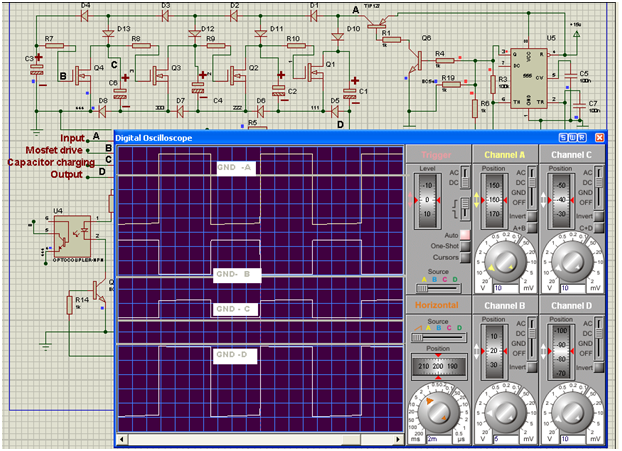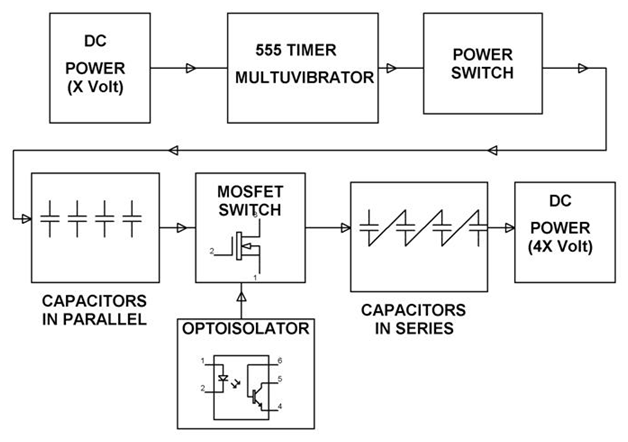# Voltage Multipliers – Classification and Block Diagram Explanation

### What are Voltage Multipliers?

Voltage multiplier refers to an electrical circuit consisting of diodes and capacitors which multiplies or increases voltage and also converts AC to DC, multiplying of voltage and rectification of current is done using voltage multiplier. Rectification of current from AC to DC is achieved by a diode and an increase in voltage is achieved by the acceleration of particles by propelling high potential produced by capacitors.

A combination of diode and capacitor makes a basic voltage multiplier circuit; AC input is given to the circuit from a power source where rectification of current and particle acceleration by capacitor gives an increased voltage DC output. The output voltage can be many times higher to input voltage so the load circuit must possess high impedance.

In this voltage doubler circuit, the first diode corrects the signal and its output is equivalent to the peak-voltage from the transformer rectified as a half-wave rectifier. An AC sign by means of the capacitor additionally achieves the second diode, and in the perspective of the DC furnished by the capacitor, this makes the output from the second diode sit on top of the first. Along these lines, the output from the circuit is double the peak-voltage of the transformer, less the diode drops.

Varieties of circuit and idea are accessible to furnish a voltage multiplier capacity of practically any variable. Applying the same rule of sitting one rectifier on top of an alternate and utilizing capacitive coupling empowers a type of step system to advance.

### Classification of Voltage Multiplier:

Classification of voltage multiplier is based on the ratio of input voltage to output voltage accordingly names also been given as

• Voltage doublers
• Voltage Tripler

#### Voltage Doubler:

The voltage doubler circuit consists of two diodes and two capacitors where each combination of diode-capacitor circuit shares positive and negative alteration also connection of two capacitors leads to double output voltage for a given input voltage.

Similarly, a each increase in a combination of diode-capacitor multiplies input voltage where voltage Tripler gives Vout = 3 Vin and voltage quadruple gives Vout = 4 Vin.

### Calculation of Output Voltage

For a voltage multiplier output voltage calculation is important considering voltage regulation and percentage ripple is important.

Vout = (sqrt 2 x Vin x N)

Where

Vout = output voltage of N stage voltage multiplier

N = no. of stages (it is no. of capacitor divided by 2).

#### Applications of Output Voltage

• Cathode Ray Tubes
• X-ray system, Lasers
• Ion pumps
• Electrostatic system
• Traveling wave tube

Example

Consider a scenario where 2.5 Kv output voltage is required with an input of 230 v, in that case, a multi-stage voltage multiplier is required in which D1-D8 gives diodes and 16 capacitors of 100 uF/400v are to be connected to achieve 2.5 Kv output.

Using formula

Vout = sqrt 2 x 230 x 16/2

= sqrt 2 x 230 x 8

= 2.5 Kv (approx)

In the above equation, 16/2 indicates no capacitors /2 gives the number of stages.

### 2 Practical Examples

#### 1. A Working Example of the Voltage Multiplier circuit to produce High voltage DC from AC signal.

The system consists of an 8 stage voltage multiplier unit.  The capacitors are used to store the charge whereas the diodes are used for rectification. As the AC signal is applied, we get a voltage across each capacitor, which approximately doubles with each stage. Thus by measuring the voltage across the 1st stage of voltage doubler and the last stage, we get the required high voltage. Since the output is a very high voltage, it is not possible to measure it using a simple multimeter. For this reason, a voltage divider circuit is used. The voltage divider consists of 10 resistors connected in series. The output is taken across the last two resistors. The obtained output is thus multiplied by 10 to get the actual output.

#### 2. Marx Generator

With the development of solid-state electronics, solid-state devices are becoming more and more suitable for pulsed power applications. They could provide the pulsed power systems with compactness, reliability, high repetition rate, and long lifetime. The rising of pulsed power generators using solid-state devices eliminates limitations of conventional components and promises pulsed power technology to be widely used in commercial applications. However, solid-state switching devices such as MOSFET or Insulated Gate Bipolar Transistor (IGBT) available now are only rated up to a few kilo Volts.

Most of the pulsed power systems demand much higher voltage ratings. Marx modulator is a unique circuit intended for voltage multiplication, as shown below. Traditionally, it employed spark gaps as switches and resistors as isolators. Therefore, it had drawbacks of low repetition rate, short lifetime, and inefficiency. In this paper, the Marx generator using solid-state devices is proposed to combine the merits of both power semiconductor switches and Marx circuits. It is designed for Plasma Source Ion Implantation (PSII)  and for the following requirements:The modern Marx generator using MOSFET

For reading the voltage and the time period please refer to the CRO screen sort.

• From the above low voltage demo unit, we find the input of 15 volts,50% duty cycle at point A goes (–Ve ) also with respect to ground. Hence a high voltage transistor has to be used for high voltage. DURING THIS TIME ALL THE CAPACITORS C1, C2, C4, C5 GET CHARGED as seen at C upto 12 volts each.
• Then through proper switching cycle C1, C2, C4, C5 get series-connected through the MOSFETs.
• Thus we get a (-Ve ) pulse voltage of  12+12+12+12=48 volts at point D

#### Application of Marx Generators – High Voltage DC by Marx generator principle

As we know by the Marx Generator principle, the capacitors are arranged in parallel to charge up and then connected to series to develop a high voltage.The system consists of a 555 timer working in an astable mode which provides an output pulse with a 50% duty cycle. The system consists of a total 4 stage multiplication stage, with each stage consisting of a capacitor, 2 diodes, and a MOSFET as a switch. The diodes are used to charge the capacitor. A high pulse from the 555 timer drives the diodes and also the optoisolators which in turn provide triggering pulses to each MOSFET. Thus the capacitors are connected in parallel as they charge up to the supply voltage. A low logic pulse from the timer results in the MOSFET switches being in off condition and the capacitors are thus connected in series. The capacitors start discharging and the voltage across each capacitor gets added, producing a voltage which is 4 times more than the input DC voltage.